# 一篇文章带你搞懂使用PID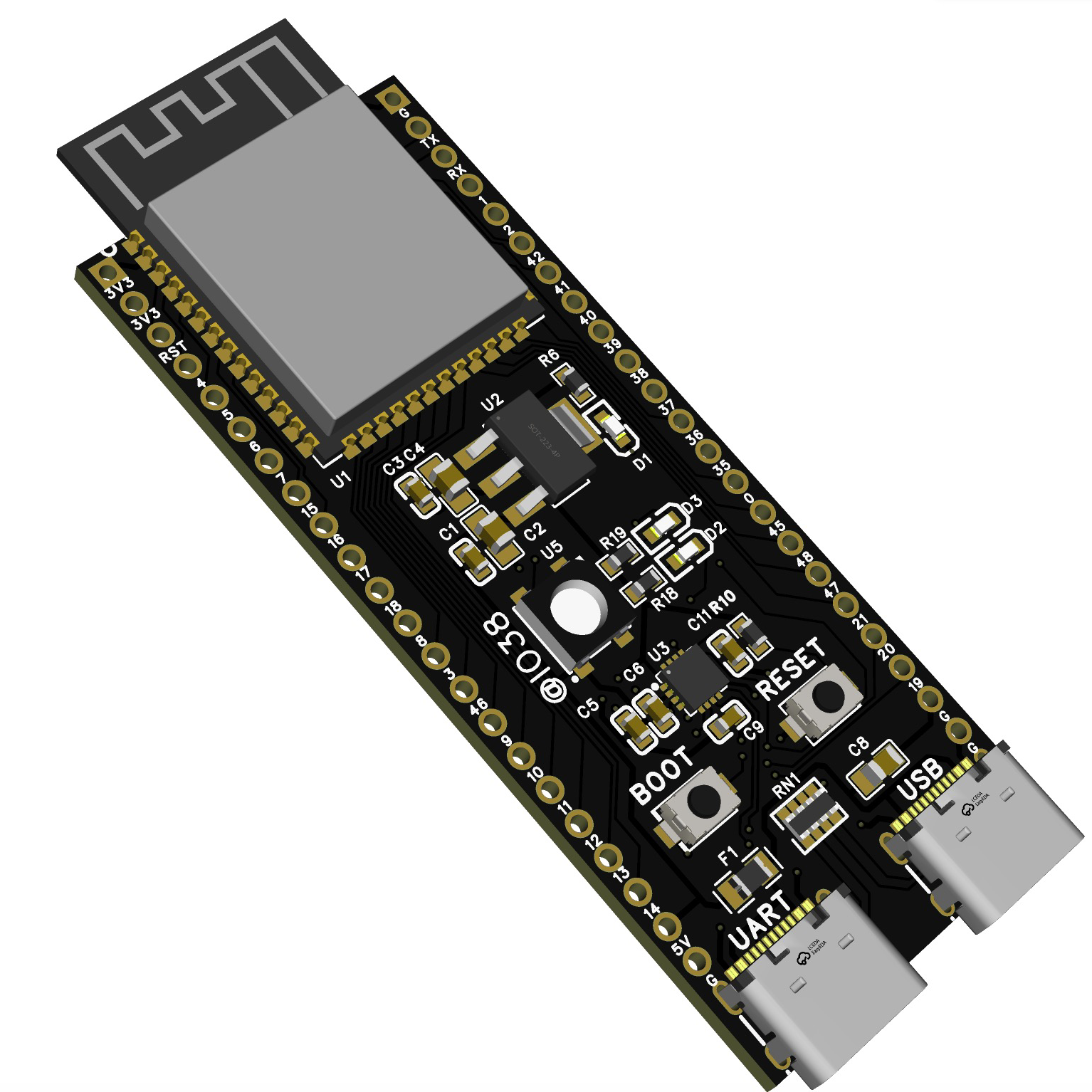zeeland 6 月前
# PID

### Introduction

• 一种是使某个物理量“保持稳定"，即便出现外界干扰也能很快回到原始的稳定状态；
• 另一种是使物理量稳定地“跟踪”给定的信号，稳定地随着给定信号变化。

• 比例环节 P 可以提高系统响应的快速性，但单独使用比例环节并不能使系统性能稳定在一个理想的状态，当有余差出现，较大的比例系数会引起较大的控制器输出，导致超调过大，系统产生振荡，使系统稳定性变差；
• 积分调节 I 可以在比例调节的基础上减小余差，提升系统的稳态性能；
• 微分环节 D 属于超前调节，可以提升系统的动态性能，使系统超调量减小、稳定性增加。

PID 中三个参数，大体对应于控制系统的三个最重要的方面：
P 对应“稳”，即稳定性，放大控制作用；I 对应“准”，消除稳态误差；D 对应“快”，对误差进行预判、做出快速反应。

### Example

#### 比例控制算法

t=1 时（表示第 1 次加水，也就是第一次对系统施加控制），那么 u=0.5*0.8=0.4，所以这一次加入的水量会使水位在 0.2 的基础上上升 0.4，达到 0.6.
**t=2 时刻**（第 2 次施加控制），当前水位是 0.6，所以 error 是 0.4。u=0.5*0.4=0.2，会使水位再次上升 0.2，达到 0.8。
t=3...
....
t=n...

``````import matplotlib.pyplot as plt

class ApplicationWithKp:
def __init__(self, ex=1, current=0.2, kp=0.5):
self.ex = ex
self.current = current
self.kp = kp
self.error = self.ex - self.current
self.iterate_times = 0

self.output_list = [self.current]
self.error_list = [self.error]

def run(self):
self.iterate(100)
self.plot()
print(self.output_list)
print("[info] iterate times: ", self.iterate_times)

def iterate(self, epoch):
""" iterate to update current, error """
for i in range(epoch):
# 加水量u
u = self.kp * self.error
self.current += u
self.output_list.append(self.current)
self.error_list.append(self.error)
self.error = self.ex - self.current
if self.current >= self.ex:
self.iterate_times = i
return
self.iterate_times = epoch

def plot(self):
l1, = plt.plot(list(range(len(self.output_list))), self.output_list, label='output')
l2, = plt.plot(list(range(len(self.error_list))), self.error_list, label='error', linestyle='--', color='r')
l3 = plt.plot(list(range(len(self.output_list))),  * len(self.output_list), linestyle='--', color='g'  )
plt.xlabel('times / s')
plt.ylabel('water volume / (m^3)')
plt.legend(handles=[l1, l2],
labels = ['output', 'error'])
plt.show()

app = ApplicationWithKp()
app.run()
``````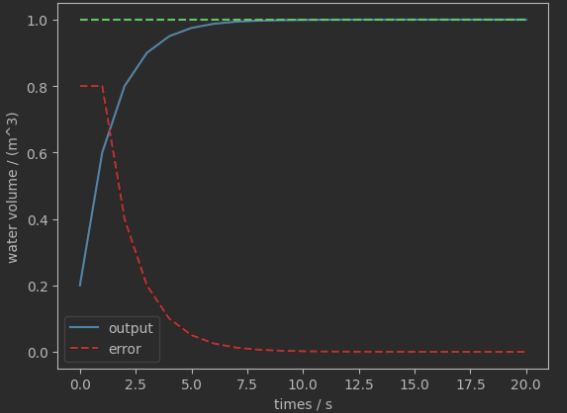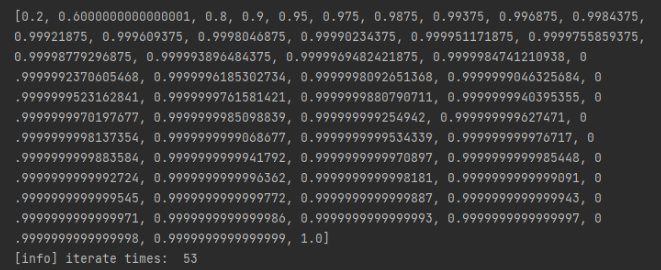``````app_2 = ApplicationWithKp(kp=0.9)
app_2.run()
``````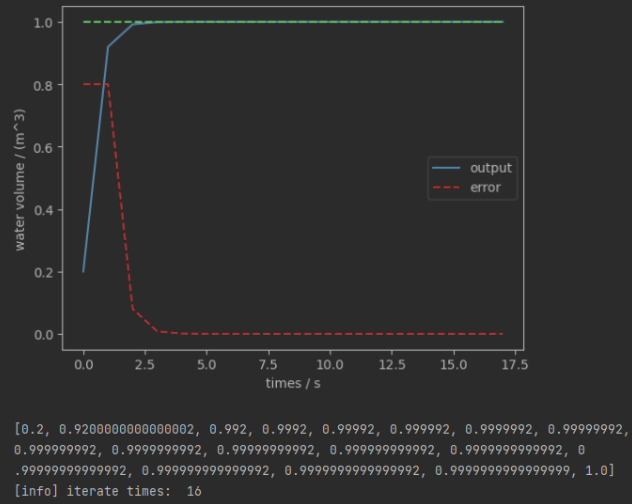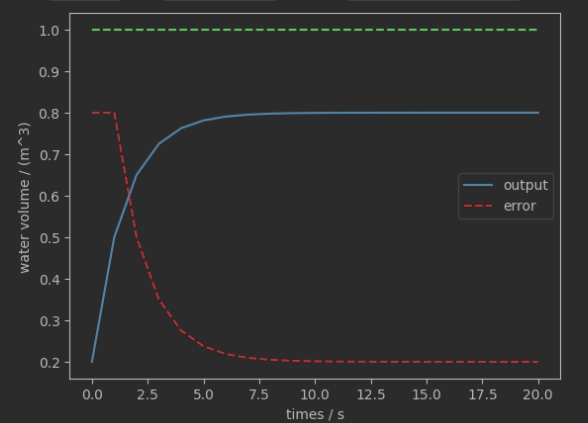#### 积分控制算法

KI 的 Python 实现，我们只需要在刚才 `ApplicationWithKp` 类的基础上稍作修改，就可以得到 `ApplicationWithKpKi` 的实现，代码如下：

``````import matplotlib.pyplot as plt

class ApplicationWithKpKi:
def __init__(self, ex=1, current=0.2, kp=0.5, ki=0.05):
self.ex = ex
self.current = current
self.kp = kp
self.ki = ki
self.error = self.ex - self.current
self.error_acc = self.error # error accumulation
self.iterate_times = 0

self.output_list = [self.current]
self.error_list = [self.error]

def run(self):
self.iterate(50)
self.plot()
print(self.output_list)
print("[info] iterate times: ", self.iterate_times)

def iterate(self, epoch):
""" iterate to update current, error """
for i in range(epoch):
# 加水量u
u = self.kp * self.error + self.ki * self.error_acc
self.current += u
self.output_list.append(self.current)
self.error_list.append(self.error)
self.error = self.ex - self.current
self.error_acc += self.error
# if self.current >= self.ex:
#     self.iterate_times = i
#     return
self.iterate_times = epoch

def plot(self):
l1, = plt.plot(list(range(len(self.output_list))), self.output_list, label='output')
l2, = plt.plot(list(range(len(self.error_list))), self.error_list, label='error', linestyle='--', color='r')
l3 = plt.plot(list(range(len(self.output_list))),  * len(self.output_list), linestyle='--', color='g'  )
plt.xlabel('times / s')
plt.ylabel('water volume / (m^3)')
plt.legend(handles=[l1, l2],
labels = ['output', 'error'])
plt.show()

if __name__ == '__main__':
app = ApplicationWithKpKi(kp=0.5, ki=0.05)
app.run()
``````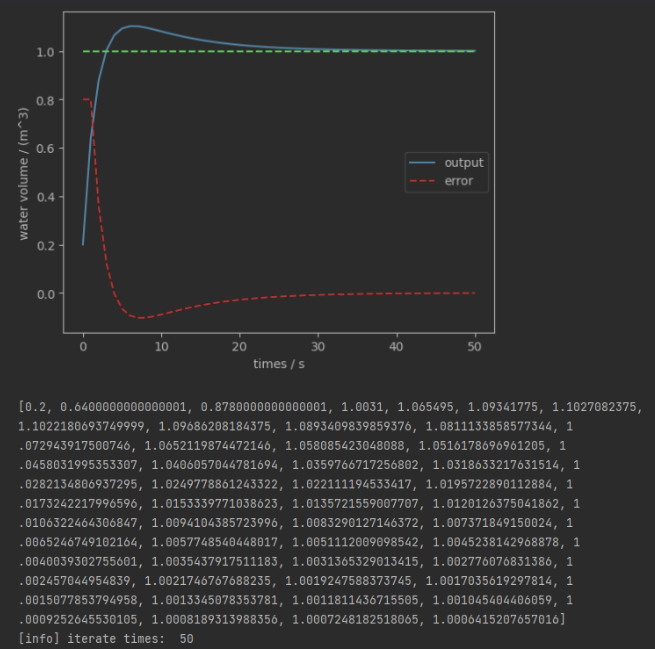#### 微分控制算法

``````import matplotlib.pyplot as plt

class ApplicationWithKpKiKd:
def __init__(self, ex=1, current=0.2, kp=0.5, ki=0.05, kd=0.1):
self.ex = ex
self.current = current
self.kp = kp
self.ki = ki
self.kd = kd
self.error = self.ex - self.current
self.error_acc = self.error # error accumulation
self.delta_error = self.error # d_e = e(t) - e(t-1)
self.iterate_times = 0

self.output_list = [self.current]
self.error_list = [self.error]

def run(self):
self.iterate(50)
self.plot()
print(self.output_list)
print("[info] iterate times: ", self.iterate_times)

def iterate(self, epoch):
""" iterate to update current, error """
for i in range(epoch):
# 加水量u
u = self.kp * self.error + self.ki * self.error_acc + self.kd * self.delta_error
self.current += u
self.error = self.ex - self.current
self.error_acc += self.error
self.delta_error = self.error - self.error_list[-1]

self.output_list.append(self.current)
self.error_list.append(self.error)
self.iterate_times = epoch

def plot(self):
l1, = plt.plot(list(range(len(self.output_list))), self.output_list, label='output')
l2, = plt.plot(list(range(len(self.error_list))), self.error_list, label='error', linestyle='--', color='r')
l3 = plt.plot(list(range(len(self.output_list))),  * len(self.output_list), linestyle='--', color='g'  )
plt.xlabel('times / s')
plt.ylabel('water volume / (m^3)')
plt.legend(handles=[l1, l2],
labels = ['output', 'error'])
plt.show()

if __name__ == '__main__':
app = ApplicationWithKpKiKd(kp=0.5, ki=0.1, kd=0.3)
app.run()
``````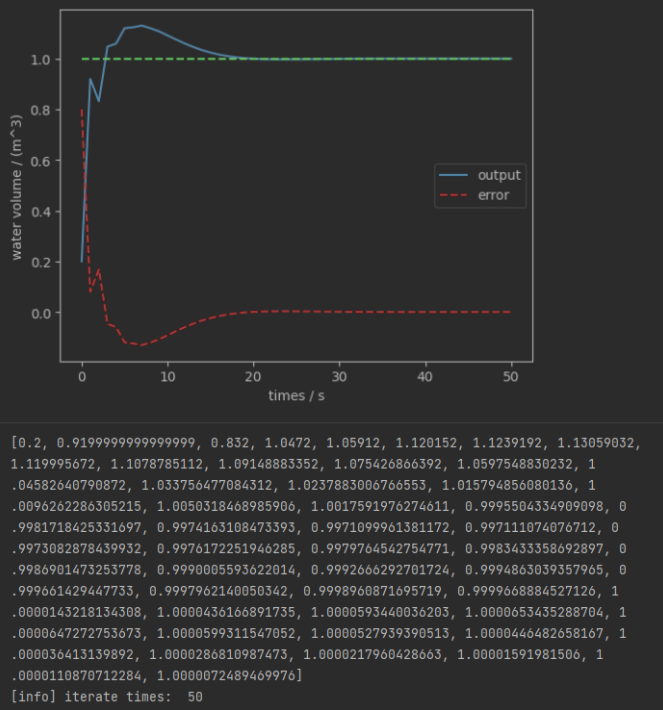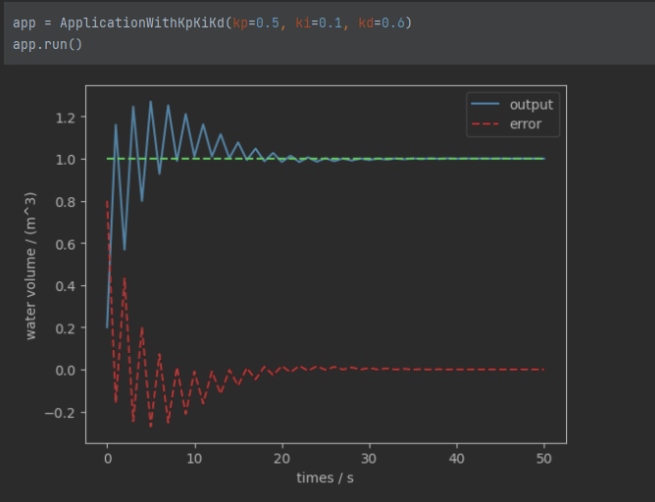### 快速上手使用 PID

``````import os
import sys
import time
import matplotlib.pyplot as plt
from simple_pid import PID

class WaterBoiler:
"""
Simple simulation of a water boiler which can heat up water
and where the heat dissipates slowly over time
"""

def __init__(self):
self.water_temp = 20

def update(self, boiler_power, dt):
if boiler_power > 0:
# Boiler can only produce heat, not cold
self.water_temp += 1 * boiler_power * dt

# Some heat dissipation
self.water_temp -= 0.02 * dt
return self.water_temp

if __name__ == '__main__':
boiler = WaterBoiler()
water_temp = boiler.water_temp

pid = PID(5, 0.01, 0.1, setpoint=water_temp)
pid.output_limits = (0, 100)

start_time = time.time()
last_time = start_time

# Keep track of values for plotting
setpoint, y, x = [], [], []

while time.time() - start_time < 10:
current_time = time.time()
dt = current_time - last_time

power = pid(water_temp)
water_temp = boiler.update(power, dt)

x += [current_time - start_time]
y += [water_temp]
setpoint += [pid.setpoint]

if current_time - start_time > 1:
pid.setpoint = 100

last_time = current_time

plt.plot(x, y, label='measured')
plt.plot(x, setpoint, label='target')
plt.xlabel('time')
plt.ylabel('temperature')
plt.legend()
if os.getenv('NO_DISPLAY'):
# If run in CI the plot is saved to file instead of shown to the user
plt.savefig(f"result-py{'.'.join([str(x) for x in sys.version_info[:2]])}.png")
else:
plt.show()
``````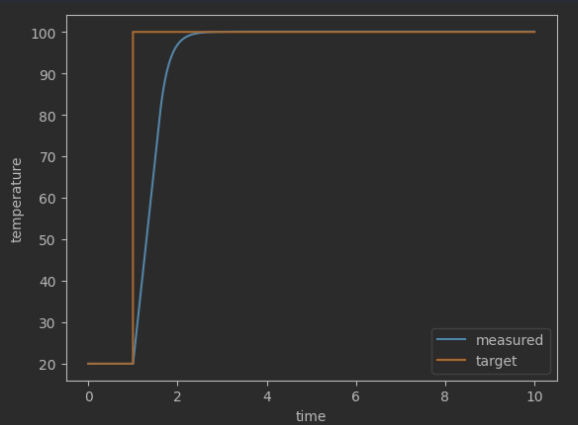zbz06 回复了 zbz06

6